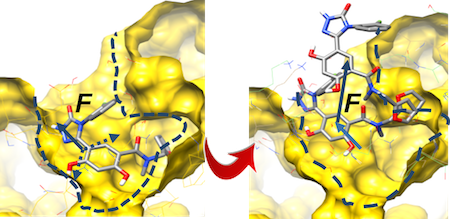### Introduction

An extension to the RAMD method for calculating relative residence times (τ) for a set of small molecules bound to a target protein.τRAMD extends the RAMD method to accelerate the egress of a set of small molecules from a target receptor. Slower dissociating molecules take longer to leave the binding pocket, or require application of a stronger force to exit within a specified simulation time. Similarly, molecules with higher dissociation rates make their exit the binding pocket more quickly, and require comparatively smaller forces for egress to occur within a defined simulation time. Thus, τRAMD may provide a computationally efficient approach to obtain relative estimates of dissociation rate constants.

Software and instructions for running RAMD and τRAMD simulations in NAMD can be found here.

and here (Tutorial).

Software and instructions for running RAMD and τRAMD simulations in Gromacs can be found here (Gromacs-RAMD).

and here (Tutorial).

References:

1. Kokh DB et. al. Estimation of Drug-Target Residence Times by τ-Random Acceleration Molecular Dynamics Simulations. J. Chem. Theory Comput. 2018; 14(7): 3859-3869 DOI: 10.1021/acs.jctc.8b00230

### Computational Tools

τ-Random Acceleration Molecular Dynamics (τRAMD) can be performed using the following software tools:

### Example Cases

For examples of previously performed studies in which τ-Random Acceleration Molecular Dynamics (τRAMD) was the primary method used, see the following example cases:

### Tutorials

The folowing tutorials describe the use of τ-Random Acceleration Molecular Dynamics (τRAMD):